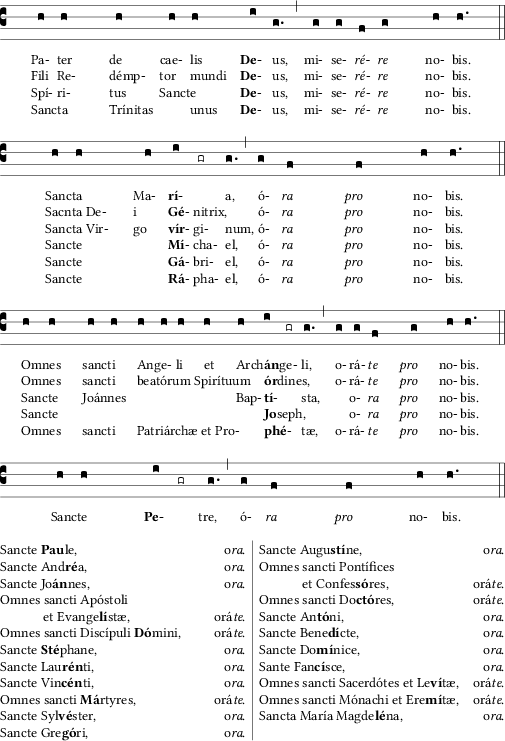# Creating a LitanyGregorio does not support multiple lines of text under the notes currently, and while it is one of our most asked for features, the coding requirements to implement it are high, making it likely to be a still distant option. However, it is possible to create something like the above litany, if you're patient and willing to work with multiple scores.

To get something like the above image, each line of music has to be coded as a separate score. Further, only the first line of the text will actually appear in the score. The rest will be added in the project file. To that end we need 4 scores to reproduce the above image. Further, to get the subsequent lines to align with the scores horizontally, we need to mark the syllables for alignment. We'll do that with a macro which we'll define in the project file:

name:Litany I;

def-m1: \grealign;
%%
(c3) Pa([em1]h)ter([em1]h) de([em1]h) cae([em1]h)lis([em1]h) <b>De</b>([em1]i)us,([em1]g.) (,)
mi([em1]g)se([em1]g)<i>ré</i>([em1]f)<i>re</i>([em1]g) no([em1]h)bis.([em1]h.) (::)

name:Litany II;

def-m1: \grealign;
%%
(c3) Sanc([em1]h)ta([em1]h) Ma([em1]h)<b>rí</b>([em1]i)([em1]gr)a,([em1]g.) (,)
ó([em1]g)<i>ra</i>([em1]f) <i>pro</i>([em1]f) no([em1]h)bis.([em1]h.) (::)


The next one is a bit tricky because there are so many notes and the tabbing environment (which is what we're going to use to align the syllables) is limited to 13 tab stops. There are ways to increase this limit, but it's easier in this case to limit ourselves to only marking some of the syllables in the first part of the line where the note stays the same.

name:Litany III;

def-m1: \grealign;
%%
(c3) Om([em1]h)nes(h) sanc([em1]h)ti(h) An([em1]h)ge(h)li(h) et(h) Arch([em1]h)<b>án</b>([em1]i)ge([em1]gr)li,([em1]g.) (,)
o([em1]g)rá([em1]g)<i>te</i>([em1]f) <i>pro</i>([em1]g) no([em1]h)bis.([em1]h.) (::)


Nota bene: In the above image the final block of lines aren't actually aligned on the score, so we don't need the alignment macro. However, we do need to introduce some extra space before the punctum cavum.

name:Litany IV;
%%
(c3) Sanc(h)te(h) <b>Pe</b>(i/gr)tre,(g.) (,)
ó(g)<i>ra</i>(f) <i>pro</i>(f) no(h)bis.(h.) (::)


Now, in the main project file we need to define the \grealign macro so that when the scores are included, LaTeX will record the position for future use. We also need to define a custom tabbing environment which will make use of those saved positions to define its tab stops. This environment can then be used to enter the subsequent lines of the litany. When put all together we get the following:

% !TEX program = LuaLaTeX+se
% !TEX encoding = UTF-8 Unicode
\documentclass{report}

\usepackage[a5paper,margin=1cm]{geometry}
\usepackage[autocompile]{gregoriotex}
\usepackage[savepos]{zref}
\usepackage{libertine}
\usepackage{multicol}

\pagestyle{empty}
\columnseprule=0.4pt

\makeatletter
\newcounter{score}
\newcounter{tabstop}[score]
\newcommand{\grealign}{%
\@bsphack%
\ifgre@boxing\else%
\kern\gre@dimen@begindifference%
\stepcounter{tabstop}%
\expandafter\zsavepos{stop-\thescore-\thetabstop}%
\kern-\gre@dimen@begindifference%
\fi%
\@esphack%
}

\newcommand{\setstops}{%
\gdef\nstabbing@stops{%
\hspace*{-\oddsidemargin}\hspace{-1in}%
\hspace*{\zposx{stop-\thescore-1} sp}\=%
}%
\count@=\@ne
\loop\ifnum\count@<\value{tabstop}%
\begingroup\edef\x{\endgroup
\noexpand\hspace{-\noexpand\zposx{stop-\thescore-\the\count@} sp}%
\noexpand\hspace{\noexpand\zposx{stop-\thescore-\the\numexpr\count@+1} sp}\noexpand\=%
}%
}\x
\repeat
\nstabbing@stops\kill
}
\makeatother

\newenvironment{nstabbing}
{\setlength{\topsep}{0pt}%
\setlength{\partopsep}{0pt}%
\tabbing%
\setstops}
{\endtabbing\stepcounter{score}}

\gresetinitiallines{0}
\gresetlastline{justified}

\begin{document}

\gregorioscore{Litany-I}%
\begin{nstabbing}
\>Fili \>Re-\>démp-\>tor \>mundi \>\textbf{De}-\>us, \>mi-\>se-\>\textit{ré}-\>\textit{re} \>no-\>bis.\\
\>Spí-\>ri-\>tus \>Sancte \>\>\textbf{De}-\>us, \>mi-\>se-\>\textit{ré}-\>\textit{re} \>no-\>bis.\\
\>Sancta \>\>Trínitas \>\>unus \>\textbf{De}-\>us, \>mi-\>se-\>\textit{ré}-\>\textit{re} \>no-\>bis.\\
\end{nstabbing}

\gregorioscore{Litany-II}
\begin{nstabbing}
\>Sacnta De-\>\>i \>\textbf{Gé}-\>nitrix, \>\>ó-\>\textit{ra} \>\textit{pro} \>no-\>bis.\\
\>Sancta Vir-\>\>go \>\textbf{ví}r-\>gi-\>num, \>ó-\>\textit{ra} \>\textit{pro} \>no-\>bis.\\
\>Sancte \>\>\>\textbf{Mí}cha-\>\>el, \>ó-\>\textit{ra} \>\textit{pro} \>no-\>bis.\\
\>Sancte \>\>\>\textbf{Gá}bri-\>\>el, \>ó-\>\textit{ra} \>\textit{pro} \>no-\>bis.\\
\>Sancte \>\>\>\textbf{Rá}pha-\>\>el, \>ó-\>\textit{ra} \>\textit{pro} \>no-\>bis.\\
\end{nstabbing}

\gregorioscore{Litany-III}
\begin{nstabbing}
\>Omnes \>sancti \>beatórum Spirítuum \>\>\textbf{ór}dines, \>\>\>o-\>rá-\>\textit{te} \>\textit{pro} \>no-\>bis.\\
\>Sancte \>Joánnes \>\>Ba-\>\textbf{ptí}-\>\>sta, \>\>o-\>\textit{ra} \>\textit{pro} \>no-\>bis.\\
\>Sancte \>\>\>\>\textbf{Jo}seph, \>\>\>\>o-\>\textit{ra} \>\textit{pro} \>no-\>bis.\\
\>Omnes \>sancti \>Patriárchæ et Proph-\>\>\textbf{é}-\>tæ, \>\>o-\>rá-\>\textit{te} \>\textit{pro} \>no-\>bis.\\
\end{nstabbing}

\gregorioscore{Litany-IV}
\begin{multicols}{2}
\noindent
Sancte \textbf{Pau}le, \hfill o\textit{ra}.\\
Sancte And\textbf{ré}a, \hfill o\textit{ra}.\\
Sancte Jo\textbf{án}nes, \hfill o\textit{ra}.\\
Omnes sancti Apóstoli\\
\hspace*{1cm} et Evange\textbf{lí}stæ, \hfill orá\textit{te}.\\
Omnes sancti Discípuli \textbf{Dó}mini,  \hfill orá\textit{te}.\\
Sancte \textbf{Sté}phane,  \hfill o\textit{ra}.\\
Sancte Lau\textbf{rén}ti,  \hfill o\textit{ra}.\\
Sancte Vin\textbf{cén}ti,  \hfill o\textit{ra}.\\
Omnes sancti \textbf{Má}rtyres,  \hfill orá\textit{te}.\\
Sancte Syl\textbf{vé}ster, \hfill o\textit{ra}.\\
Sancte Gre\textbf{gó}ri,  \hfill o\textit{ra}.\\
Sancte Augu\textbf{stí}ne, \hfill o\textit{ra}.\\
Omnes sancti Pontífices\\
\hspace*{1cm} et Confes\textbf{só}res, \hfill orá\textit{te}.\\
Omnes sancti Do\textbf{ctó}res, \hfill orá\textit{te}.\\
Sancte An\textbf{tó}ni, \hfill o\textit{ra}.\\
Sancte Bene\textbf{dí}cte, \hfill o\textit{ra}.\\
Sancte Do\textbf{mí}nice, \hfill o\textit{ra}.\\
Sante Fan\textbf{cí}sce, \hfill o\textit{ra}.\\
Omnes sancti Sacerdótes et Le\textbf{ví}tæ, \hfill orá\textit{te}.\\
Omnes sancti Mónachi et Ere\textbf{mí}tæ, \hfill orá\textit{te}.\\
Sancta María Magde\textbf{lé}na, \hfill o\textit{ra}.\\
\end{multicols}

\end{document}


You'll notice that our definition of \grealign contains two of the GregorioTeX internals: \ifgre@boxing and \gre@dimen@begindifference. The first is needed to prevent the creation of spurious tab stops. In order to align the syllable text (that it knows about) and the notes, GregorioTeX puts the notes into a box first so that it can measure them. However, since it's only putting them into a box, and not placing that box on the page, when GregorioTeX does this, the position of the notes is 0, which is not a number we want to save. We only want to save the position when they are being placed on the page: i.e. when we're not boxing (hence the immediate \else).

The other internal, \gre@dimen@begindifference is the travel from the beginning of the notes to the beginning of the text. By following that travel before we save our position, we force the tab stop to be aligned with the text, rather than the notes. Of course, once we've saved the stop position, we have to undo that move so that GregorioTeX can continue to create the score with proper alignment.

Finally, after running LuaLaTeX on the document twice (once to store the positions, the second to use them), we get something like this:Obviously this isn't identical to the original, mostly because I've made a best guess at the page size, but in some ways it improves on it because I make better use of the page size I've chosen. Each line of music, for instance, stretches all the way across the page, allowing me to make the alignment of the lines clearer.

4.1.0.

5.1.0.